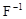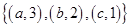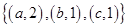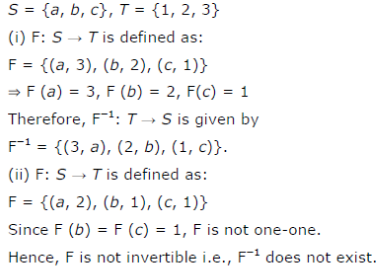# Let S = F-1 and T = {1, 2, 3}.Find F-1 of the following functions F from S to T, if it exist

Let S =and T = {1, 2, 3}. Findof the following functions F from S to T, if it exists.
(i) F =(ii) F =